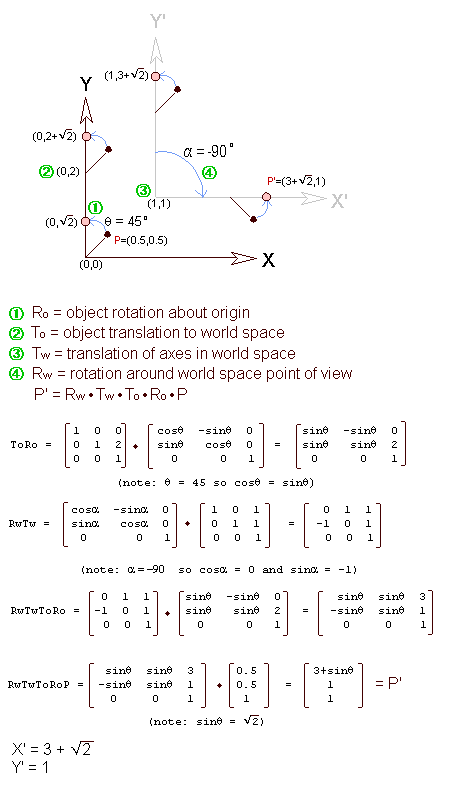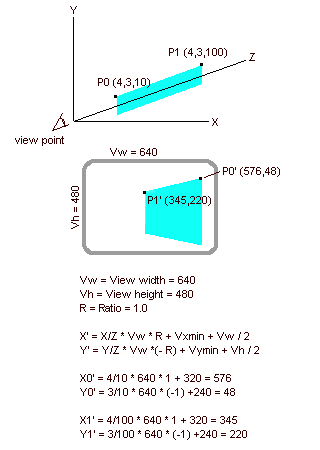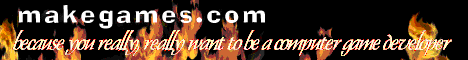# Fastgraph 3D Tutorial

## Appendix 1: Doing the Math

### Rotation and Translation

In the Chapter 2 we defined rotation and translation. For the purpose of writing 3D games, we usually want to rotate an object around its center of gravity, and then view it from some position in world space. This is a 4 step process which must be done in order, as follows:

(1) Rotate the point about its object space origin.
(2) Translate the object space origin to a fixed position in world space.
(3) Translate the world space coordinates to the viewpoint.
(4) Rotate the world space coordinates about the viewpoint.

(Note: you don't need to know this because Fastgraph does the matrix math internally. This example is provided because some people find it interesting.)

For simplicity in drawing, I am going to work the problem in 2D XY space. The concepts are easily expanded to 3D space.

The Problem. Suppose we define a point (x,y) at (0.5, 0.5) in object space. In other words it is half a unit away from its center of gravity in both the positive X and Y directions. Suppose further that this point's center of gravity will reside at a world space location of (0,2.0). Suppose we want to rotate this object one quarter turn around its own center of gravity. Then we move our point of view to (1,1) in world space, and rotate our view by -90 degrees. What are the coordinates of P', the translated point?Fig. A1.1 Example Rotation/Translation problem.

 Look at Figure A1.1. There are 4 steps in the process: two rotations and two translations. The first thing that happens is the rotation around the object's center of gravity. Since the object is defined in its own object space, this rotation takes place before any other translations or rotations. We rotate by angle alpha=45 degrees. The result is labeled (1) above. The next thing that happens is the translation to the object's position in world space. This is a fixed location. It is where the object will always be. The chair will always be in the corner of the room, for example. The translation from object space to world space is labeled (2). The next thing that happens is the world space translation. This is where we move our point of view, by moving every point in the world by a fixed amount. In this case, the origin is moved to the point (1,1) so 1 is added to the X and Y of every point in the world, including our point P. This step is labeled (3). An interesting but non-intuitive feature of step 3 is that moving the origin to (1,1) is equivalent to moving our point of view to (-1,-1). Finally, we rotate 1/2 turn in the clockwise direction around our new point of view. This step is labeled (4). This produces the desired result. The matrix multiplications are not difficult. If you have a background in math, you should have no trouble seeing how it works. If you are unfamiliar with matrix dot products, you need to take a class in linear algebra. The important properties to remember are matrix multiplications are associative: (AB)C = A(BC) and they are not commutative: AB != BA. The composition of the rotation and translation matrices is discussed in Foley/Van Dam, Chapter 5. A final note: The transformation matrix, T, which is the product of RwTwToRo, may be used for all the points with the same center of gravity. If you have an object like a chair which has many surfaces that are rotated together, you can save considerable time by calculating the matrix T once, and then applying it to all the points using the formula P' = TP. This is exactly what fg_3Dpov() and fg_3Dsetobject() do. The fg_3Dpov() function calculates RwTw and the fg_3Dsetobject() function calculates ToRo and then combines it with RwTw to create RwTwRoTo. This last matrix is automatically applied whenever you draw a surface with fg_3Dpolygonobject() or fg_3Dtexturemapobject().

### Projection

Compared to rotation and translation, projection is easy. There are no matrices involved, just some simple algebra. The formulas are shown in Figure 3.2, along with the projection of some sample points.Fig. A1.2 Sample Projection.

 Notice that the farther away a point is in the z direction, the closer it is to the center of the viewport. As points approach infinity, their coordinates become centered. This makes sense. Think of looking at the moon. If you stare right at it, it will be centered in your vision. Things that are close look more spread out. Look at your computer screen. If you stare straight at the center of the screen, the right edge of the screen extends to the right edge of your vision, and the left edge of the screen is at the left edge of your vision. The Vxmin, Vymin, Vw, Vh, and R variables are set in fg_3Dviewport(). The projections are handled automatically, along with the rotation and translation, in functions like fg_3Dpolygon().

### More Rotation information